College of Surveying and Geo-Informatics, Shandong Jianzhu University, Jinan 250101, China

Telchina Smart Industry Group Company Limited, Jinan 250101, China

Corresponding Author Email:
caifei@sdjzu.edu.cn
Page:
35-42
|
DOI:
https://doi.org/10.18280/isi.240104
8 December 2018
|
Accepted:
3 February 2019
|
Published:
20 April 2019
| Citation

OPEN ACCESS

Abstract:

Link prediction for complex networks is a research hotspot. The main purpose is to predict the unknown edge according to the structure of the existing network. However, the edges in real-world networks are often sparsely distributed, and the number of unobserved edges is usually far greater than that of observed ones. Considering the weak performance of traditional link prediction algorithms under the above situation, this paper puts forward a novel link prediction algorithm called network adjacency matrix blocked-compressive sensing (BCS). Firstly, the diagonal blocks were subjected to sparse transformation with the network adjacency matrix; Next, the measurement matrix was rearranged into a new measurement matrix using the sorting operator; Finally, the subspace pursuit (SP) algorithm was introduced to solve the proposed algorithm. Experiments on ten real networks show that the proposed method achieved higher accuracy and consumed less time than the baseline methods.

Keywords:

compressive sensing (CS), measurement matrix, adjacency matrix, link prediction, subspace pursuit (SP)

1. Introduction

Link prediction for complex networks has become a research hotspot in recent years. The purpose is to identify unobserved edges in the existing network or forecast future edges based on the current network structure . Link prediction has been applied to recommend friends or interest points in social networks , predict the possible protein interactions without costly experiments in biology , simulate the drug interactions through a limited number of tests in medical pharmacy , and analyze the structure of criminal and terrorist networks to combat organized crime . The existing methods for link prediction are either based on similarity or grounded on probability.

The similarity-based methods  assume that two nodes with a high similarity are more likely to be edged, and characterize the similarity between different nodes by local and global indices. The common indices include common neighbors (CN) index , adamic-adar (AA) index , local path (LP) index , and resource allocation (RA) index . The methods using local indices enjoy fast speed and high efficiency. However, their prediction is not sufficiently accurate, as the node similarity is only constrained by local information. By contrast, the methods using global indices, e.g. Katz index , consumes lots of time to process large networks, despite its good accuracy.

The probability-based methods often assume that the network has a known structure. Under this assumption, the model is built and the parameters are estimated by statistical methods. The common probability-based models are hierarchical structure model  and random block model . These methods boast many advantages in network analysis. However, the parameter learning and reasoning greatly increase the computing complexity, which limits the application range of such methods.

Both the similarity- and probability-based methods predict the possibility of unobserved edges between network nodes according to the structure of the existing network. Nevertheless, many real networks are so sparse that the edges of observed nodes only account for a small portion of network edges . This poses a huge challenge to the existing link prediction methods, calling for the improving the link prediction in sparse network.

A possible way of improvement lies in the compressive sensing (CS) theory. Since it was proposed by Donoho , the CS has been implemented mainly in the processing of signals and images. The basic idea is that, in a proper low-dimensional representation, the information needed for a signal is fully contained in its under-sampled data. In other words, a signal can be reconstructed from a small set of sampled data, which is often the case in real networks with sparse edges. Considering the poor effect of existing link prediction methods in sparse network, this paper puts forward a novel link prediction algorithm called network adjacency matrix blocked-compressive sensing (BCS). Firstly, the diagonal blocks were subjected to sparse transformation with the network adjacency matrix; Next, the measurement matrix was rearranged into a new measurement matrix using the sorting operator; Finally, the subspace pursuit (SP) algorithm was introduced to solve the proposed algorithm. Experiments on ten real networks show that the proposed method achieved higher accuracy and consumed less time than the baseline methods.

2. Problem Statement and Proposed Method

2.1 Introduction to the CS

In the CS theory , the measurement $x\in {{R}^{n}}$ can be defined as:

${{x}_{m\times 1}}={{A}_{m\times n}}{{b}_{n\times 1}}$ (1)

where m<<n; A is the measurement matrix; $b\in {{R}^{n}}$ is the original signal. The sparsest solutions can be obtained by:

$\underset{b}{\mathop{\min }}\,||b|{{|}_{0}}\ \ s.t.\ \ Ab=x$ (2)

Since it is an NP-hard problem, (2) can be solved by l1-minimization:

$\underset{b}{\mathop{\min }}\,||b|{{|}_{1}}\ \ s.t.\ \ Ab=x$ (3)

From (2) and (3), we have:

$\underset{b}{\mathop{\min }}\,||b|{{|}_{1}}+||Ab-x||_{2}^{2}$ (4)

Here, (4) is known as the least absolute shrinkage and selection operator (LASSO). After introducing the sparseness control parameter $\lambda$, (4) can be rewritten as:

$\hat{b}=\arg \underset{b}{\mathop{\min }}\,\lambda ||b|{{|}_{1}}+||Ab-x||_{2}^{2}$ (5)

The construction of measurement matrix is shown in Figure 1 below.

figure_1.jpgFigure 1. Construction of measurement matrix

2.2 Problem statement

In graph theory, an undirected network can be described as G=(V, E), where V and E are the set of nodes and set of edges, respectively. In the network, the number of nodes and the number of edges can be denoted as N=|V| and M=|E|, respectively. Let X be the adjacency matrix of the network. If there are edges between nodes i and j, then xij=xji=1; otherwise, xij=xji=0.

For each dataset, the network edges were divided into a training set Etrain and a test set Etest. Apparently, Etrain∪Etest=E and Etrain∩Etest =∅. Let Xtrain and Xtest be the adjacency matrix of the training set and the test set, respectively. Both matrices consist of 1 or 0 elements. Assuming that L=|Etest| is the number of edges in the test set, then the number of edges in the training set can be expressed as |Etrain|=M-L. In addition, the number of all possible edges in the network is denoted as the candidate set $\left| \overline{E} \right|=\frac{N(N-1)}{2}-(M-L)$. During the solution, the model was trained with the training set Etrain, each possible edge between the nodes in the candidate set were given a score, and the scores were ranked in descending order. In this case, the node pair on the top has the largest probability of edge connection. Then, the test set Etest results were verified by different evaluation metrics.

2.3 Proposed method

Let X be a $c\times r$ adjacency matrix of the network. First, the matrix was divided into n $B\times B$ matrix blocks, and the column vector of the i-th block was labelled xi, where i=1, 2, 3, …, n, $n=c\times r/{{B}^{2}}$. Under the same conditions, xi was measured by measurement matrix ${{\phi }_{B}}$. Then, the vector of the measured value yi was obtained, where the vector length is MB(MB<<B2). The vector yi can be expressed as:

${{y}_{i}}={{\phi }_{B}}{{x}_{i}}\ \ (i=1,2,3,...,n)$ (6)

where the ${{M}_{B}}\times {{B}^{2}}$ matrix ${{\phi }_{B}}$ is a Gaussian matrix. The original adjacency matrix x can obtain m CS values. For the original adjacency matrix, the total measurement matrix $\phi$ is the block diagonal matrix. The values of diagonal elements ${{\phi }_{B}}$ can be expressed as:

$\phi =\left[ \begin{matrix} {{\phi }_{B}} & {} & {} & {} & {} & {} & {} \\ {} & {{\phi }_{B}} & {} & {} & {} & {} & {} \\ {} & {} & {{\phi }_{B}} & {} & {} & {} & {} \\ {} & {} & {} & {{\phi }_{B}} & {} & {} & {} \\ {} & {} & {} & {} & . & {} & {} \\ {} & {} & {} & {} & {} & . & {} \\ {} & {} & {} & {} & {} & {} & {{\phi }_{B}} \\\end{matrix} \right]$. (7)

The above analysis shows that the total measurement matrix $\phi$ can be stored with ${{M}_{B}}\times {{B}^{2}}$ matrix by our method, rather than with the $B\times B$ matrix. The required storage space is very small when B is small, which accelerate the implementation.

In our method, the matrix is solved by l1-norm :

${{\hat{b}}_{i}}=\arg \underset{{{b}_{i}}}{\mathop{\min }}\,\lambda ||{{b}_{i}}|{{|}_{1}}+||A{{b}_{i}}-{{x}_{i}}||_{2}^{2}$

$s.t.\ {{y}_{i}}={{\phi }_{B}}{{x}_{i}}={{\phi }_{B}}\psi {{\hat{b}}_{i}}\ \ i=1,2,3,...,n$ (8)

where $\psi$ is the transformation matrix. To explain the principle of CS with matrix block, the network in Figure 2(a) was cited as an example. The construction of the measurement matrix is illustrated in Figure 2 below.

figure_2.jpgFigure 2. Construction of measurement matrix

The network adjacency matrix X in Figure 2 can be expressed as:

X=\left[ \begin{array}{*{35}{r}} 0 & 1 & 1 & 0 & 0 \\ 1 & 0 & 1 & 1 & 1 \\ 1 & 1 & 0 & 1 & 1 \\ 0 & 1 & 1 & 0 & 1 \\ 0 & 1 & 1 & 1 & 0 \\\end{array} \right]=\left[ \begin{align} & {{x}_{1}}\ \ \ {{x}_{3}} \\ & {{x}_{2}}\ \ \ {{x}_{4}} \\ \end{align} \right] (9)

where xi are matrix blocks. According to Figure 2, the variable y can be described as:

eq10.jpgSorting operator R, the vector (x1, x2, x3, x4)T can be rearranged into adjacent matrix:

\left[ \begin{align} & {{x}_{1}} \\ & {{x}_{2}} \\ & {{x}_{3}} \\ & {{x}_{4}} \\ \end{align} \right]=R\cdot X (11)

The following can be derived from (10) and (11):

$y=\phi R\cdot X=\phi \psi \cdot X$   (12)

Thus, the similarity adjacency matrix ${{x}^{*}}\in {{R}^{N}}$ can be obtained :

${{X}^{*}}=\arg \underset{{{x}_{i}}}{\mathop{\min }}\,\lambda ||{{x}_{i}}|{{|}_{1}}+||\phi \psi {{x}_{i}}-{{y}_{i}}||_{2}^{2}$

$s.t.\ \ \ {{y}_{i}}=\phi {{x}_{i}}={{\phi }_{B}}\psi x_{i}^{*}\ \ i=1,2,3,...,n$ (13)

The l1-norm minimization cannot guarantee the nonnegativity of the solution, but the size of network adjacency matrix must be nonnegative. To solve the problem, the SP algorithm [16, 17] was employed to recalibrate the prediction results.

Taking edges as the unknown vectors, a linear system was formed to find a solution to link prediction. The proposed method can identify unobserved edges in the existing network or forecast future edges based on the current network structure. The details on our method are given below.

Algorithm 1 BCS algorithm

 Input: Undirected network G=(V, E) Measurement matrix $\phi \in {{R}^{M\times N}}$ Vector $y\in {{R}^{M}}$ Network adjacency matrix $x\in {{R}^{N}}$ Sparsity K for network adjacency matrix Output: Similarity adjacency matrix ${{X}^{*}}\in {{R}^{N}}$ Steps: 1. Initialize error r0=y, index set ${{\wedge }_{0}}=\phi$, and number of iterations n=1; 2. Generate intermediate candidate vectors ${{u}_{n}}={{\phi }^{T}}{{r}_{n-1}}$; 3. Judge reliability: ${{\wedge }_{n}}=\sup (({{u}_{n}}){{|}_{K}})$, find the index with maximum K; 4. Update index: ${{\overline{\Lambda }}_{n}}={{\Lambda }_{n}}\bigcup {{\Lambda }_{n-1}}$ 5. Update intermediate candidate vectors: ${{\theta }_{n}}={{\phi }_{\overline{{{\Lambda }_{n}}}}}y$; 6. Update candidate vectors: ${{\widehat{\theta }}_{n}}=({{\theta }_{n}}){{|}_{K}}$; 7. Update error: ${{r}_{n}}=y-\phi {{\theta }_{n}}$ 8. Update the number of iteration n=n+1; If n<

3. Evaluation Metrics and Data Resource

3.1 Evaluation metrics

Two evaluation metrics, namely, area under the curve (AUC)  and precision , were set up to compare the performance of our method with that of baseline methods. The two metrices are defined as follows.

(1) AUC

The AUC index measures the overall accuracy of the algorithm. It can be understood as the probability that a randomly selected missing edge has a higher score than a randomly chosen non-existent edge out of all unobserved edges. The AUC can be expressed as:

$AUC=\frac{{{n}^{'}}+{{n}^{''}}}{n}$ (14)

where n is the number of independent comparisons; n’ is that the probability estimate of randomly selecting an edge in the n’ test set is greater than that of randomly selecting an edge in the E test set; n’’ is that the probability estimate of randomly selecting an edge in the n” test set equals that of randomly selecting an edge in the nonexistent edge set.

If all the scores are generated from an independent, identical distribution, the AUC value will approximate 0.5. Therefore, AUC>0.5 shows how much the algorithm outperforms random selection.

(2) Precision

Precision is defined as the ratio of the most likely predicted accuracy in the first L predicted edges given by the algorithm:

$Precision=\frac{{{L}_{r}}}{L}$ (15)

where L is the size of the predicted edges; Lr is the size of correctly predicted edges. Obviously, the higher the precision, the better the accuracy.

3.2 Baseline algorithms

The performance of our algorithm was compared with that of six typical similarity algorithms, i.e. the baseline algorithms, namely, common neighbors (CN) , resource allocation (RA) , adamic-adar (AA) , preferential attachment (PA) , local path (LP)  and Katz 10]. These similarity indices are described in details in Table 1.

Table 1. Six classical similarity indices

 Name Score Description CN $S_{xy}^{CN}=\left| \Gamma (x)\bigcap \Gamma (y) \right|$ $\Gamma (x)$ and $\Gamma (y)$ are the sets of neighbors of node x and node y, respectively. RA $S_{xy}^{RA}=\sum\nolimits_{z\in \Gamma (x)\bigcap \Gamma (y)}{\frac{1}{{{k}_{z}}}}$ kz is the degree of node z. AA $S_{xy}^{AA}=\sum\nolimits_{z\in \Gamma (x)\bigcap \Gamma (y)}{\frac{1}{\log {{k}_{z}}}}$ kz is the degree of node z. PA $S_{xy}^{PA}={{k}_{x}}{{k}_{y}}$ kx is degree of node x. LP $S_{xy}^{LP}={{({{A}^{2}}+\alpha {{A}^{3}})}_{xy}}$ α is the adjustable parameter, A is the adjacency matrix Katz $S_{xy}^{Katz}={{((I-\beta {{A}^{-1}})-I)}_{xy}}$ β is the adjustable parameter, I is the diagonal matrix

3.3 Experiment data

Table 2. The statistics of the real-world networks

 |V| |E| C r H FoodWeb 128 2075 0.335 -0.112 32.422 1.237 Karate 34 78 0.571 -0.476 4.588 1.693 Jazz 198 2742 0.617 0.020 27.697 1.395 USAir 332 2126 0.625 -0.208 12.807 3.464 Neural 297 2148 0.292 -0.163 14.465 1.801 Metabolic 453 2025 0.646 -0.226 8.940 4.485 Email 1133 5451 0.220 0.078 9.622 1.942 PB 1490 16715 0.263 -0.221 22.436 3.622 Yeast 2361 6646 0.130 -0.099 5.630 2.944 Router 5022 6258 0.012 -0.138 2.492 5.503

Note: |V| and |E| are the set of nodes and the set of edges, respectively; C is the clustering coefficient; r is the degree-degree correlation coefficient; <k> is the average degree. H is the degree heterogeneity.

To verify the performance of our algorithm, ten real networks from different domains were selected for experiments, including FoodWeb , Karate , Jazz , USAir , Neural , Metabolic , Email , PB , Yeast  and Router . The statistics on the real-world networks are listed in Table 2 above.

4. Results and Analysis

As mentioned before, our method was compared with six classical method through experiments on ten typical networks. Firstly, the observed edges were randomly divided into a training set and a test set. The training set was used to establish the prediction model, while the test set was adopted to verify the link prediction accuracy in complex networks. Next, the typical networks were transformed into undirected networks. Tables 3 and 4 compare the AUC and precision, two metrics of prediction accuracy, of the contrastive algorithms, respectively. Both the AUCs and precisions are the average values of 100 runs. The optimal AUC or precision on the ten networks are bolded, and the proportion of training set is 90%.

Table 3. The AUCs of different methods

 Network BCS CN AA RA PA LP Katz FoodWeb 0.8152 0.6104 0.6094 0.6120 0.7342 0.6223 0.6745 Karate 0.8116 0.6994 0.7341 0.7283 0.7008 0.7209 0.7371 Jazz 0.9663 0.9545 0.9612 0.9701 0.7668 0.9591 0.9485 USAir 0.9599 0.9355 0.9474 0.9537 0.8856 0.9427 0.9242 Neural 0.8847 0.8441 0.8589 0.8644 0.7529 0.8595 0.8575 Metabolic 0.9319 0.9198 0.9506 0.9544 0.8174 0.9243 0.9197 Email 0.8973 0.8442 0.8464 0.8467 0.7779 0.8974 0.8942 PB 0.9336 0.9361 0.9392 0.9393 0.9327 0.9495 0.9500 Yeast 0.8412 0.7061 0.7066 0.7061 0.7865 0.8357 0.8184 Router 0.6654 0.5580 0.5579 0.5579 0.4694 0.6320 0.3738

Table 4. The precisions of different methods

 Network BCS CN AA RA PA LP Katz FoodWeb 0.1762 0.0707 0.0755 0.0754 0.1607 0.0758 0.1023 Karate 0.1487 0.1525 0.1538 0.1538 0.0863 0.1750 0.1613 Jazz 0.6225 0.5041 0.5242 0.5391 0.1304 0.5126 0.4920 USAir 0.3905 0.3730 0.3898 0.4505 0.3164 0.3738 0.3695 Neural 0.1262 0.0962 0.1039 0.1025 0.0575 0.0981 0.1027 Metabolic 0.2113 0.1378 0.1932 0.2680 0.0999 0.1449 0.1408 Email 0.1503 0.1392 0.1552 0.1401 0.0174 0.1467 0.1355 PB 0.0861 0.1729 0.1716 0.1493 0.0652 0.1735 0.1744 Yeast 0.1070 0.0924 0.0932 0.0741 0.0093 0.0950 0.0935 Router 0.0253 0.0168 0.0162 0.0098 0.0096 0.0214 0.0227

It can be seen that the proposed BCS outperformed the baseline methods under the AUC metric in 6 out of the 10 networks, namely, FoodWeb, Karate, USAir, Neural, Yeast and Router. Under the precision metric, our method outperformed the other methods in 7 out of the 10 networks, including FoodWeb, Jazz, Neural, Metabolic, Email, Yeast and Router. In particular, our method achieved the best values in large sparse networks like Yeast and Router.

The AUC and precision values of all the seven methods in the ten networks were further compared using different proportions of training set. The proportion of the training set was changed from 0.5 to 0.9. The results in Figures 3 and 4 show that our method achieved the best prediction accuracy and robustness among all methods.

figure_3a.jpg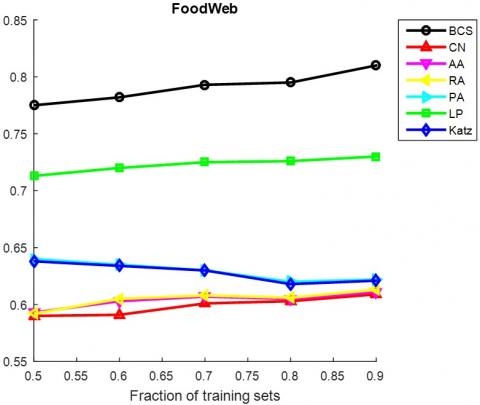(a) FoodWeb

figure_3b.jpg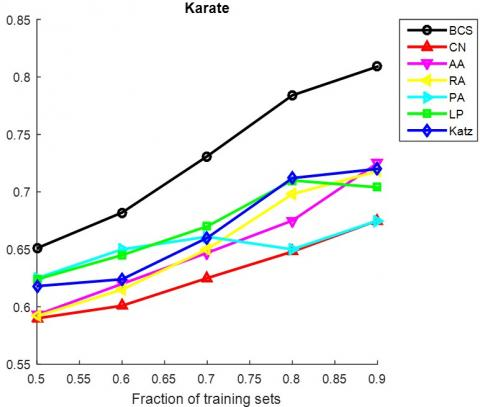(b) Karate

figure_3c.jpg(c) Jazz

figure_3d.jpg(d) Neural

figure_3e.jpg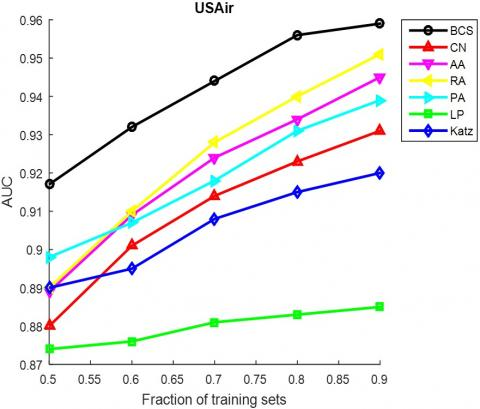(e) USAir

figure_3f.jpg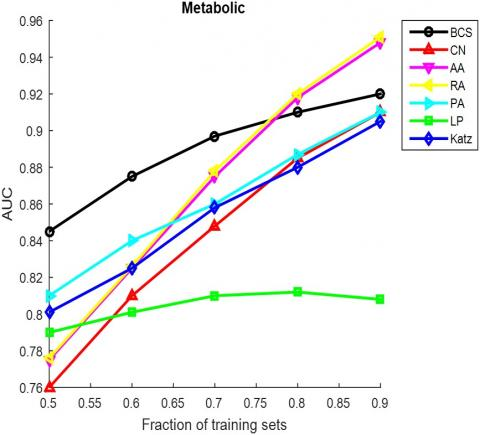(f) Metabolic

figure_3g.jpg(g) Email

figure_3h.jpg(h) PB

figure_3i.jpg(i) Yeast

figure_3j.jpg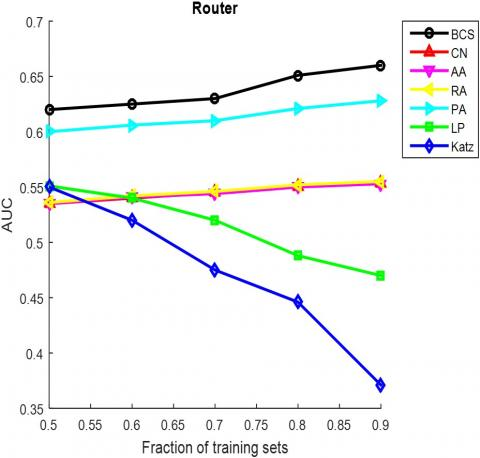(j) Router

Figure 3. The AUCs of different methods under different proportions of training set

figure_4a.jpg(a) FoodWeb

figure_4b.jpg(b) Karate

figure_4c.jpg(c) Jazz

figure_4d.jpg(d) Neural

figure_4e.jpg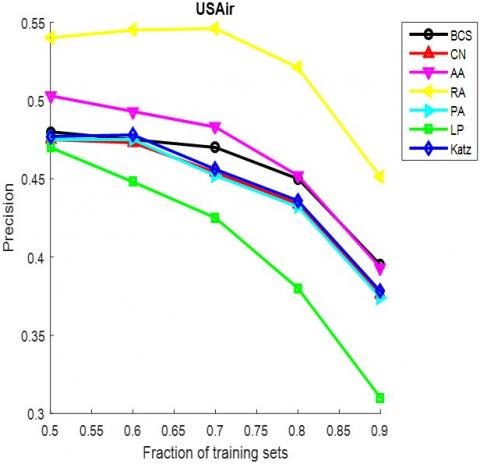(e) USAir

figure_4f.jpg(f) Metabolic

figure_4g.jpg(g) Email

figure_4h.jpg(h) PB

figure_4i.jpg(i) Yeast

figure_4j.jpg(j) Router

Figure 4. The precisions of different methods under different proportions of training set

Next, the computing time of our method was contrasted against that of the other methods through several experiment on a workstation with 64GB RAM and 3.10GHz 8-core processor. The comparison result is shown in Figure 5 below.

figure_5.jpgFigure 5. Comparison of computing time of different methods

As shown in Figure 5, the proposed method consumed much less time in computation than most of the contrastive methods. This proves the validity and reliability of our method.

5. Conclusions

Real-world networks are often very sparse, posing a severe challenge to traditional link prediction methods. What is worse, these methods usually have high complexity and low accuracy. To overcome these problems, this paper designs the BCS method for link prediction in complex networks, which uses the sorting operator to transform the measurement matrix into a new measurement matrix. The performance of the proposed method was verified by two evaluation metrics, the AUC and precision, through experiments on ten classical networks. The results show that our method outperformed the baseline methods in accuracy and computing time. Suffice it to say that this research designs a feasible, effective and competitive way to predict edges in complex networks.

Acknowledgement

This work was supported by the National Natural Science Foundation of China (41601489), the Housing and Urban Rural Development Science and Technology Project of Shandong Province (2018-K2-03), University Science and technology project of Shandong Province (J18KB088), National College Students Innovation and Entrepreneurship Training project (201710430050).

References

 Xie R, Shao ZF, Liu B, Xu SB. (2018). Application of asymmetric information in link prediction. Journal of Computer Applications 38(6): 1698-1702, 1708. https://doi.org/10.11772/j.issn.1001-9081.2017102467

 Shahmohammadi A, Khadangi E, Bagheri A. (2016). Presenting new collaborative link prediction methods for activity recommendation in Facebook. Neurocomputing, 210: 217-226. https://doi.org/10.1016/j.neucom.2016.06.024

 Li Y, Zhang XF, Yi M, Liu YY. (2015). Link prediction for the gene regulatory network. Acta Mathematica Scientia 35(5): 1018-1024. https://doi.org/10.3969/j.issn.1003-3998.2015.05.017

 Fokoue A, Hassanzadeh O, Sadoghi M, Zhang P. (2016). Predicting drug-drug interactions through similarity-based link prediction over web data. In International Conference Companion on World Wide Web, 2016: 175-178. https://doi.org/10.1007/978-3-319-34129-3_47

 Fan C, Liu Z, Lu X, Xiu BX, Chen Q. (2017). An efficient link prediction index for complex military organization. Physica A: Statistical Mechanics and its Applications 469: 572-587. https://doi.org/10.1016/j.physa.2016.11.097

 Lorrain F, White HC. (1971). Structural equivalence of individuals in social networks. The Journal of Mathematical Sociology 1(1): 49-80. https://doi.org/10.1080/0022250X.1971.9989788

 Adamic LA, ADAR E. (2003). Friends and neighbors on the Web. Social Networks 25(3): 211-230. 10.1016/S0378-8733(03)00009-1

 Lv L, Jin CH, Zhou T. (2009). Similarity index based on local paths for link prediction of complex networks. Physical Review E, Statistical, Nonlinear, and Soft Matter Physics 80(4): 046122. https://doi.org/10.1103/physreve.80.046122

 Zhou T, Lv L, Zhang YC. (2009). Predicting missing links via local information. European Physical Journal B, 71(4): 623-630. https://doi.org/10.1140/epjb/e2009-00335-8

 Katz L. (1953). A new status index derived from sociometric analysis. Psychometrika 18(1): 39-43. https://doi.org/10.1007/BF02289026

 Clauset A, Moore C, Newman ME. (2008). Hierarchical structure and the prediction of missing links in networks. Nature 453(7191): 98-101. https://doi.org/10.1038/nature06830

 Guimerà R, Sales-Pardo M. (2009). Missing and spurious interactions and the reconstruction of complex networks. Proceedings of the National Academy of Sciences 106(52): 22073-22078. https://doi.org/10.1073/pnas.0908366106

 Costa M, Desmarais BA, Hird JA. (2016). Science use in regulatory impact analysis: The effects of political attention and controversy. Review of Policy Research 33(3): 251-269. https://doi.org/10.1111/ropr.12171

 Dnonho DL. (2006). Compressed sensing. IEEE Transactions on Information Theory 52(4): 1289-1306. https://doi.org/10.1109/TIT.2006.871582

 Meng J, Li H, Han Z. (2009). Sparse event detection in wireless sensor networks using compressive sensing. 2009 43rd Annual Conference on Information Sciences and Systems, pp. 181-185. https://doi.org/10.1109/CISS.2009.5054713

 Lee H, Lee DS, Kang H. (2011). Sparse brain network recovery under compressed sensing. IEEE Transactions on Medical Imaging 30(5): 1154-1165. https://doi.org/10.1109/TMI.2011.2140380

 Liben-Nowell D, Kleinbergl J. (2003). The link prediction problem for social networks. Journal of the American Society for Information Science and Technology 58(7): 556-559. https://doi.org/10.1002/asi.20591

 Hanley JA, Mcneil BJ. (1982). The meaning and use of the area under a receiver operating characteristic (ROC) curve. Radiology 143(1): 29-36. https://doi.org/10.1148/radiology.143.1.7063747

 Herlocker JL, Konstan JA, Terveen LG, Riedl, JT. (2004). Evaluating collaborative filtering recommender systems. ACM Transactions on Information Systems 22(1): 5-53. https://doi.org/10.1145/963770.963772

 Barabasi AL, Albert R. (1999). Emergence of scaling in random networks. Science 286(5439): 509-509. https://doi.org/10.1126/science.286.5439.509

 Ulanowiza RE, Bondavalli C, EGNOTOVICH MS. (1998). Network analysis of trophic dynamics in South Florida ecosystem, FY 97: The Florida Bay Ecosystem.

 Zachary WW. (1977). An information flow model for conflict and fission in small groups. Journal of Anthropological Research 33(4): 452-473. https://doi.org/10.1086/jar.33.4.3629752

 Gleiser PM, Danon L. (2003). Community structure in jazz. Advances in Complex Systems 6(4): 565-573. https://doi.org/10.1142/S0219525903001067

 Batagelj V, Mrvar A. (2006). Pajek datasets.

 White JG, Southgate E, Thomson JN, Brenner S. (1986) The structure of the nervous system of the nematode Caenorhabditis elegans. Philosophical Transactions of the Royal Society of London B: Biological Sciences 1(3): 314-340. https://doi.org/10.1098/rstb.1986.0056

 Duch J, Arenas A. (2005). Community detection in complex networks using extremal optimization. Physical Review E Statistical Nonlinear and Soft Matter Physics 72(2): 027104. https://doi.org/10.1103/PhysRevE.72.027104

 Guimerà R, Danon L, Díaz-Guilera A, Giralt F, Arenas, A. (2003). Self-similar community structure in a network of human interactions. Physical Review E 68(6): 065103. https://doi.org/10.1103/PhysRevE.68.065103

 Adamic LA, Glance N. (2005). The political blogosphere and the 2004 U.S. election: Divided they blog. In Proceedings of the 3rd International Workshop on Link Discovery, New York, NY, USA, pp. 36-43. https://doi.org/10.1145/1134271.1134277

 von Mering C, Krause R, Snel B, Cornell M, Oliver SG, Fields S, Bork P. (2002). Comparative assessment of large-scale data sets of protein-protein interactions. Nature 417(6887): 399-403. https://doi.org/10.1038/nature750

 Spring N, Mahajan R, Wetherall D. (2002). Measuring ISP topologies with rocket fuel. In Proceedings of the 2002 Conference on Applications, Technologies, Architectures, and Protocols for Computer Communications, New York, NY, USA, pp. 133-145.